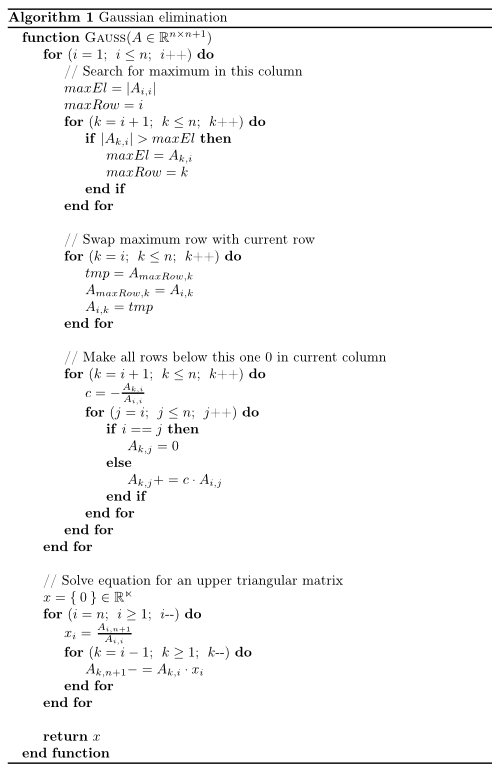### Problem Set 1

You can think of vectors representing many dimensions of related information. For instance, Netflix might store all the ratings a user gives to movies in a vector. This is clearly a vector of very large dimensions (in the millions) and very sparse as the user might have rated only a few movies. Similarly, Amazon might store the items purchased by a user in a vector, with each slot or dimension representing a unique product and the value of the slot, the number of such items the user bought. One task that is frequently done in these settings is to find similarities between users. And, we can use dot-product between vectors to do just that. As you know, the dot-product is proportional to the length of two vectors and to the angle between them. In fact, the dot-product between two vectors, normalized by their lengths is called as the cosine distance and is frequently used in recommendation engines.

1. Calculate the dot product u.v where u = [0.5; 0.5] and v = [3; −4]
u <- c(0.5, 0.5)
v <- c(3, -4)

product <- sum(u*v)
print(product)
##  -0.5
1. What are the lengths of u and v? Please note that the mathematical notion of the length of a vector is not the same as a computer science definition.

Length of u:

len.u <- sqrt(sum(u^2))
print(len.u)
##  0.7071068

Length of v:

len.v <- sqrt(sum(v^2))
print(len.v)
##  5
1. What is the linear combination: 3u − 2v?
lc = 3*u - 2*v
print(lc)
##  -4.5  9.5
1. What is the angle between u and v?
theta <- acos(product / (len.u * len.v))
print(theta)
##  1.712693

### Problem Set 2

Set up a system of equations with 3 variables and 3 constraints and solve for x. Please write a function in R that will take two variables (matrix A & constraint vector b) and solve using elimination. Your function should produce the right answer for the system of equations for any 3-variable, 3-equation system. You don’t have to worry about degenerate cases and can safely assume that the function will only be tested with a system of equations that has a solution. Please note that you do have to worry about zero pivots, though. Please note that you should not use the built-in function solve to solve this system or use matrix inverses. The approach that you should employ is to construct an Upper Triangular Matrix and then back-substitute to get the solution. Alternatively, you can augment the matrix A with vector b and jointly apply the Gauss Jordan elimination procedure.

matrix <- rbind(c(1,1,3),c(2,-1,5),c(-1,-2,4))
vector <- c(1,2,6)

aug <- cbind(matrix,vector)

print(aug)
##              vector
## [1,]  1  1 3      1
## [2,]  2 -1 5      2
## [3,] -1 -2 4      6

To solve this question, I used the following algorithm, from this site.solve_matrix <- function(matrix){
n = nrow(matrix)
for (i in (1:n)){
max <- abs(matrix[i,i])
max.row <-  i
if (i+1 <= n) {
for (k in ((i+1): n)){
if (abs(matrix[k,i]) > max){
max = abs(matrix[k,i])
max.row <- k
}
}
}
# Swap maximum row with current row (column by column)
for (k in (i: (n+1))){
tmp = matrix[max.row,k]
matrix[max.row,k] = matrix[i,k]
matrix[i,k] = tmp
}

# Make all rows below this one 0 in current column
if (i+1 <= n) {
for (k in ((i+1): n)){
c = -matrix[k,i]/matrix[i,i]
for (j in (i: (n+1))){
if (i == j){
matrix[k,j] = 0
}
else{
matrix[k,j] = matrix[k,j] + (c * matrix[i,j]  )
}
}
}
}
}
# Solve equation Ax=b for an upper triangular matrix A
x = c(1:n)*0
for (i in (n:1)){
x[i] = matrix[i,(n+1)]/matrix[i,i]
for (k in ((i-1): 1)){
matrix[k,(n+1)] = matrix[k,(n+1)] - matrix[k,i] * x[i]
}
}
return(x)
}

solution <- solve_matrix(aug)
print(solution)
##  -1.5454545 -0.3181818  0.9545455

The solution above reflects the solution of x = [-1.55, -0.32, 0.95].